# Multiplying multi digit numbers

Here you will learn how to multiply multi digit numbers with strategies, such as the relationship between addition and subtraction, the properties of operations, and how to use the standard algorithm.

Students will first learn about multiplying multi digit numbers in 4 th grade and learn to use the standard algorithm to multiply in 5 th grade.

## What is multiplying multi digit numbers?

Multiplying multi digit numbers is multiplying large numbers together and finding their product.

When first working with multi-digit multiplication, the focus is on using strategies including place value understanding, properties of operations and the relationship between multiplication and division.

For example,

20 \times 61= \; ?

• I know that 10 \times 61 = 610, so I can double that to find 20 \times 61.

610 + 610 = 1,220

• I know that 61 = 30 + 30 + 1.

Since 30 \times 20 = 600 and 1 \times 20 = 20. This means…

\begin{aligned} & 20 \times 61 \\ & =20 \times(30+30+1) \\ & =600+600+20 \\ & =1,220 \end{aligned}

• I can use an area model to solve.

Notice there are many ways to solve the same problem – using partial products, using the distributive property and area models. Knowing the names of each strategy is not important, but understanding why they work and making connections between them is.

After students have had sufficient practice understanding division with mostly single-digit numbers in 3 rd grade and multi-digit whole numbers in 4 th, the standard algorithm is taught in 5 th grade.

For example,

20 \times 61= \; ?

Start by writing the column multiplication equation. Leave an empty row at the top.

First, multiply the top number by the ones place in the bottom number (multiplier).

This shows that 20 \times 1=20

Then multiply the top number by the tens place of the bottom number (multiplier).

Since the 6 in 61 represents 60, we place a 0 in the ones position as a place holder.

This shows that 60 \times 20=1,200

Notice that since multiplying 6 \times 2=12 produces 2 digits in the product, this step requires regrouping. You record the 2 and regroup the 1 to the next position – in this case the thousands.

So 20 \times 61=1,220.

Note: This page does not cover multiplication with decimals. However, students use strategies in fifth grade to multiply decimals up to hundredths and use the standard algorithm in 6th grade to multiply all decimals.

### What is multiplying multi digit numbers?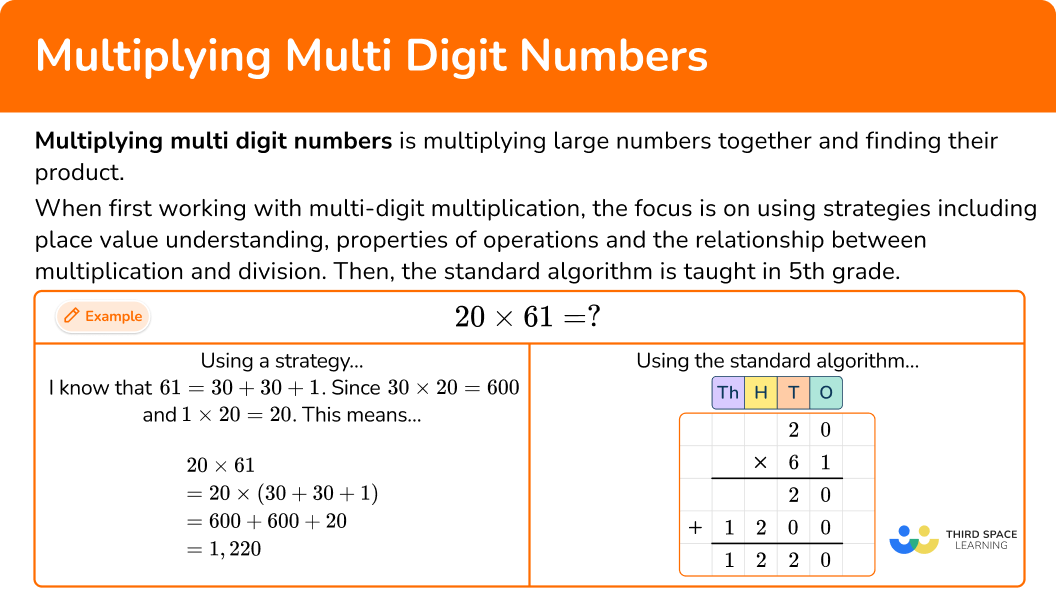## Common Core State Standards

How does this apply to 4 th grade math and 5 th grade math?

• Grade 4: Numbers and Operations in Base 10 (4.NBT.B.5)
Multiply a whole number of up to four digits by a one-digit whole number, and multiply two two-digit numbers, using strategies based on place value and the properties of operations. Illustrate and explain the calculation by using equations, rectangular arrays, and/or area models.

• Grade 5: Numbers and Operations in Base 10 (5.NBT.B.5)
Fluently multiply multi-digit whole numbers using the standard algorithm.

## How to multiply multi digit numbers with strategies

In order to multiply multi digit numbers with strategies:

1. Write the multiplication equation.
2. Choose a solving strategy.
3. Solve the equation.

## Multiplying multi digit numbers examples

### Example 1: two digit by one digit

Solve 19 \times 8

1. Write the multiplication equation.

19 \times 8= \; ?

2Choose a solving strategy.

19 \times 8 is one group of 8 less than 20 \times 8. You can use this related fact to solve.

3Solve the equation.

20 \times 8=160 and 160-8=152.

So 19 \times 8=152.

### Example 2: three digit by one digit

Solve 238 \times 6.

Write the multiplication equation.

Choose a solving strategy.

Solve the equation.

### Example 3: double-digit multiplication

There are 81 classroom novel sets. Each novel set has 34 books. How many books are there in all the sets?

Write the multiplication equation.

Choose a solving strategy.

Solve the equation.

## How to multiply multi digit numbers with the standard algorithm

In order to multiply multi digit numbers with the standard algorithm:

1. Write the column multiplication equation.
2. Multiply the top number by the ones position of the bottom number (multiplier) – recording the partial product.
3. Repeat for each position in the multiplier.
4. Add all the partial products together.

### Example 4: multiplying a two-digit number by 1 digit multiplier

Solve 34 \times 7 using the standard algorithm.

Write the column multiplication equation.

Multiply the top number by the ones position of the bottom number (multiplier) – recording the partial product.

Repeat for each position in the multiplier.

Add all the partial products together.

### Example 5:

Solve 281 \times 5 using the standard algorithm.

Write the column multiplication equation.

Multiply the top number by the ones position of the bottom number (multiplier) – recording the partial product.

Repeat for each position in the multiplier.

Add all the partial products together.

### Example 6:

Solve 37 \times 19 using the standard algorithm.

Write the column multiplication equation.

Multiply the top number by the ones position of the bottom number (multiplier) – recording the partial product.

Repeat for each position in the multiplier.

Add all the partial products together.

### Teaching tips for multiplying multi digit numbers

• Before multiplying with larger numbers, students should have had plenty of opportunities to learn multiplication of 1- digit numbers. Preferably, students will have memorized their multiplication facts, before moving on to 2- digit multiplication and beyond, but this is not a requirement. You can provide a multiplication table to students who are still mastering their basic facts.

• Emphasize students making sense of multiplication problems over speed related activities. This includes encouraging students to show their work, solve in more than one way, compare their strategies to others, and use estimation or other strategies to consider if their answer is reasonable.

• While math worksheets and multi-digit multiplication worksheets can be useful for this skill, make sure to expose students to all types of multiplication problems; ones that provide many different contexts and opportunities to solve in different ways. This includes using a mixture of word problems, projects, real world scenarios, calculator activities, etc. throughout your lesson plans.

• As students are learning the algorithm, provide them with a step by step guide and/or graphic organizer that explains each part. This gives them something to refer back to and can help them make sense of the algorithm.

### Easy mistakes to make

• Thinking only one multiplication strategy or algorithm will work
When using strategies, students can solve problems with related facts, properties of numbers, partial products, area models and more. Additionally, even though one standard algorithm is taught, there are others. Some students may be familiar with the Lattice algorithm or others.

When learning to multiply large numbers, many students use the partial products method. For this method to be successful, students need to keep track of all the partial products and add them together at the end. If a student’s work is messy or incomplete, it can lead to mistakes.

• Not using place value when solving
Place value should always be considered when solving – no matter the strategy or algorithm. This helps prevent mistakes and can be used to identify whether or not an answer is reasonable.

• Thinking that the top number has to be larger than the bottom
For example,
34 \times 815 can be set up either way:

While the setup on the right is the most common, both will work.

### Practice multiplying multi digit numbers

1. Solve 88 \times 3.

48270264244You can use a related fact to solve.

88 \times 3 is two groups of 3 less than 90 \times 3.

90 \times 3=270

and

270-3-3=264.

So 88 \times 3=264.

2. Solve 801 \times 9.

7,209817298,100Break up 801 into parts \rightarrow 801=800+1.

Since ​​ 800 \times 9=7,200 and 1 \times 9=9. This means…

\begin{aligned} & 801 \times 9 \\\\ & =(800+1) \times 9 \\\\ & =(800 \times 9)+(1 \times 9) \\\\ & =7,200+9 \\\\ & =7,209 \end{aligned}

3. Each recipe calls for 18 ounces of fruit. Jeylani wants to make 33 recipes. How many ounces of fruit does she need?

54594297300Use an area model to solve.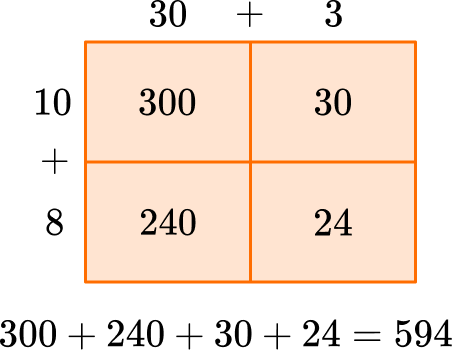4. Solve 76 \times 4 with the algorithm.

2842,82452304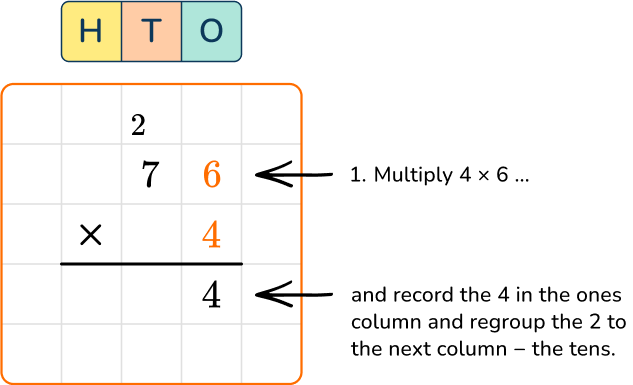Notice that since multiplying 6 \times 4=24 produces 2 digits in the product, this step requires regrouping.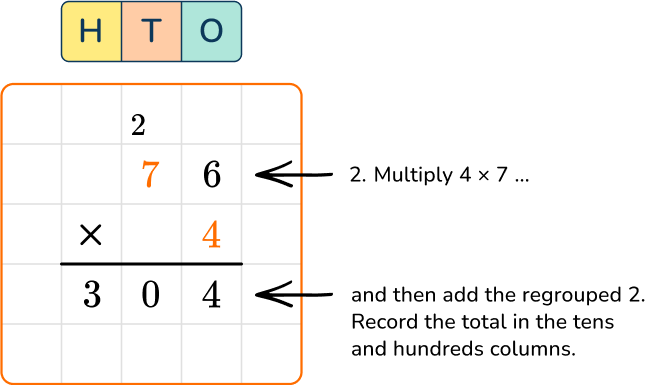Notice that we added the regrouped 2 after multiplying: 4 \times 8=28 and 28 + 2 = 30.

5. Solve 925 \times 4 with the algorithm.

2,7803,7003,6003,780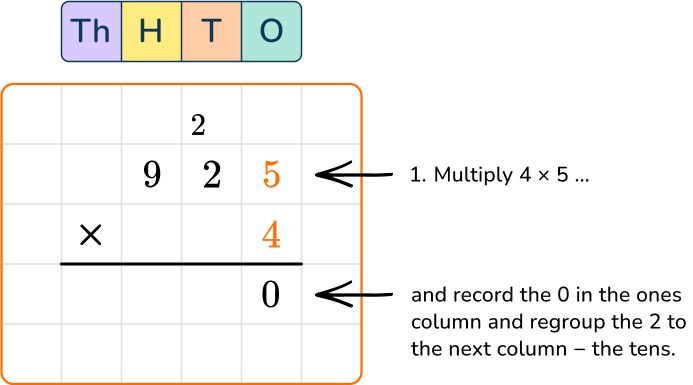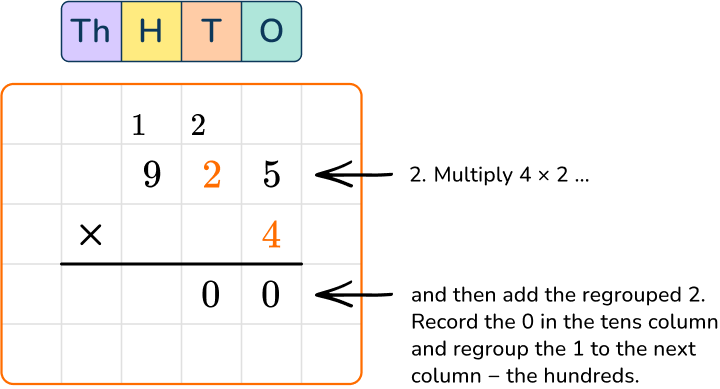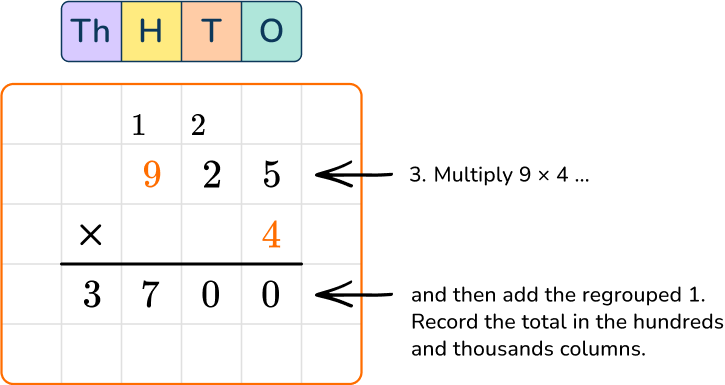6. An English teacher requires his students to read 45 minutes each night. How many total minutes will each student have read after 41 nights?

1,8451,6452251,800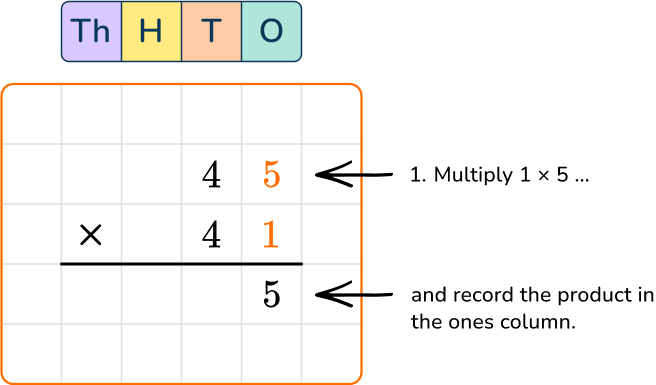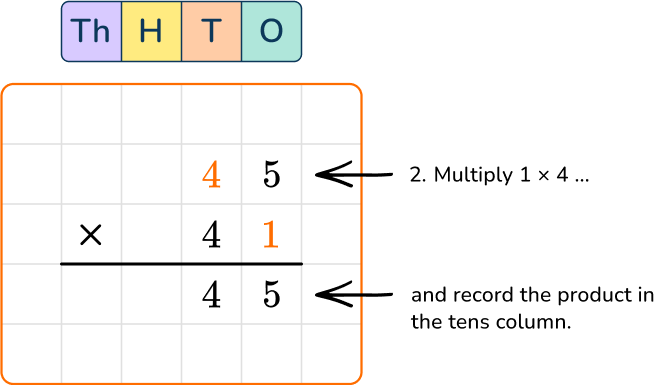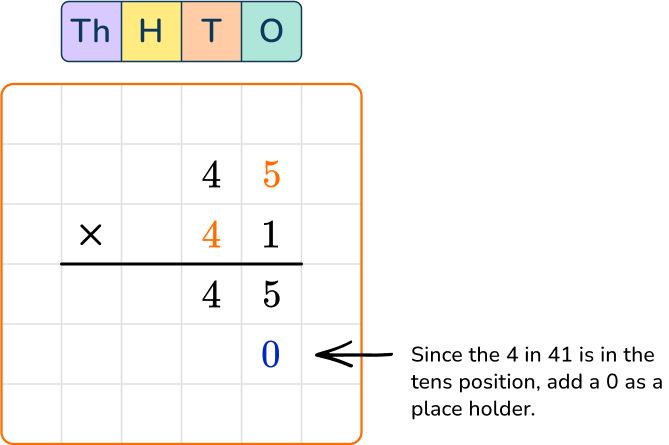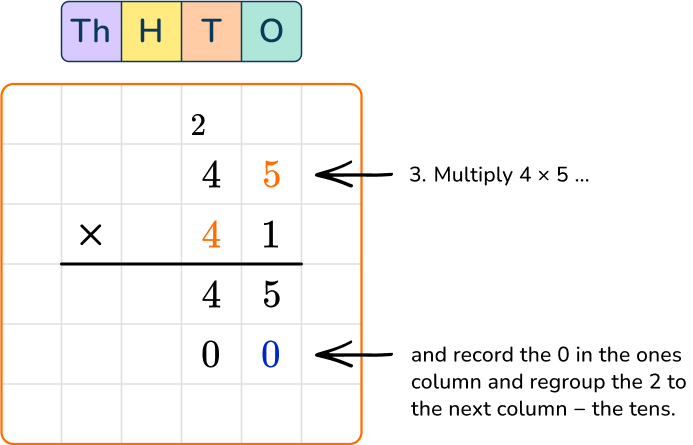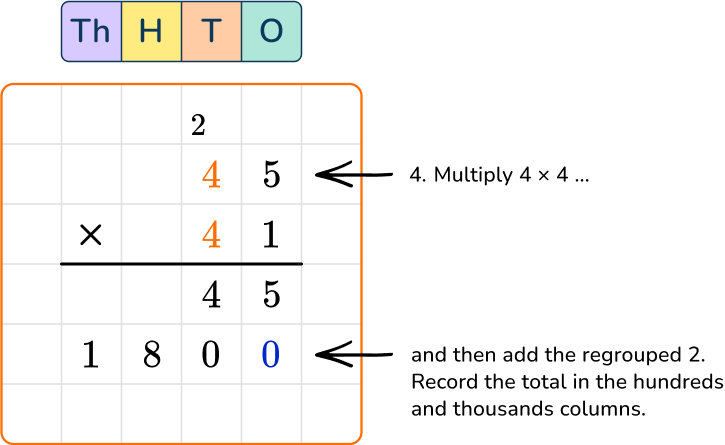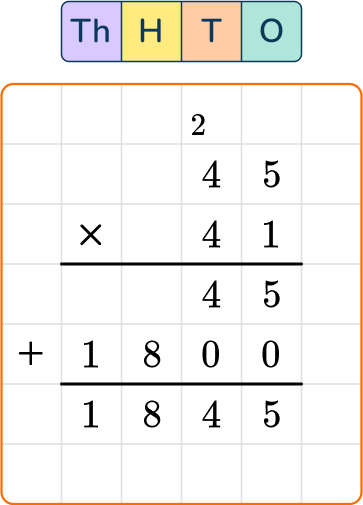## Multiplying multi digit numbers FAQs

What is the learning progression for multiplying whole numbers?

In third grade, students work with multiplying one-digit numbers within 100. Their work with multiplication is directly connected to their work with division.

Students extend their work in fourth grade to multi-digit multiplication by multiplying 2- digit numbers, 3- digit numbers and 4- digit numbers.

In 5 th grade, they learn how to solve problems with the standard algorithm. In all three grade levels, students use their reasoning and understanding of numbers and multiplication to solve.

It is important to note that there are additional standards where students focus on how to multiply by 10, 100, etc., through place value understanding. This work comes before learning how to multiply with other larger numbers.

When do students learn to multiply with decimal numbers and fractions?

Students begin multiplying fractions by whole numbers in 4 th grade, in both mathematical and real world contexts. Students expand to multiply fractions by fractions in 5 th grade – using strategies and algorithms.

This is also when they begin to multiply with decimals – but only using strategies and place value understanding. Students learn the algorithm to multiply with decimals in 6 th grade.

## Still stuck?

At Third Space Learning, we specialize in helping teachers and school leaders to provide personalized math support for more of their students through high-quality, online one-on-one math tutoring delivered by subject experts.

Each week, our tutors support thousands of students who are at risk of not meeting their grade-level expectations, and help accelerate their progress and boost their confidence.